## finding the number of diagonals in a polygon

How many diagonals are there in an octagon?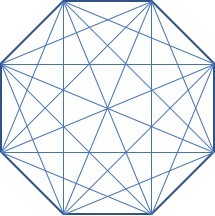Instead of trying to count them all, use the formula for the number of diagonals in any polygon:$d= \dfrac{n(n-3)}{2}$

where n = the number of sides and d= the number of diagonals. For an octagon, n= 8, so$d= \dfrac{8(8-3)}{2} = 20$

This is a good formula to memorize and its derivation is pretty simple, so let’s see it. We will use the same octagon diagram, but only draw the diagonals from one vertex: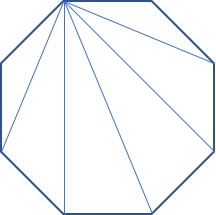You can see that from this vertex on the upper left, a diagonal can be drawn to every vertex except the two adjacent vertices and to itself. So the number of diagonals from any vertex in an n-gon is (n-3). Since there are n vertices, the total number of diagonals is n(n-3). But we’ve just counted every diagonal twice (once from each endpoint), so to find the number of different diagonals, we divide by two. This gives us the formula above.

## finding internal or external angles of regular polygons

How do you find the measure of an internal angle or an external angle on a regular polygon? It’s quite easy, if you memorize two formulas. All you have to do is count the number of sides on the polygon and plug it into the formula.

For internal angles, use the formula$\alpha = \dfrac{180(n-2)}{n}$

For external angles, the formula is even easier:$\beta = \dfrac{360}{n}$

For example, here is a regular octagon, so n = 8. The internal angle is labeled α and the external angle is β.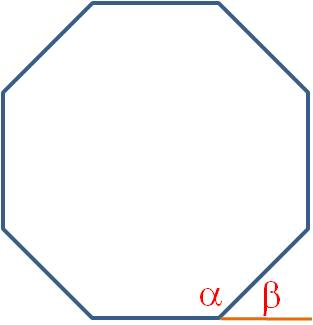$\alpha = \dfrac{180(8-2)}{8}=135 \textdegree$$\beta = \dfrac{360}{8} = 45 \textdegree$

And here’s a trick to save you some time: You can see in the figure that the internal and external angles are always supplementary. (This is true in any polygon, even if it isn’t regular.) So if you need to find the internal angle, you can find the external angle instead with the simpler formula. Then the supplement to that angle is the internal angle!

## Divisibility rules

You always need to simplify fractions and radicals before you box your final answer. In order to simplify these, you need to be able to factor integers. There was a teacher at my middle school who urged his students to “learn your gozintas!” In other words, know whether a number goes into the number you are factoring. Now that calculators are so common, many students today don’t think they need to learn their gozintas. However, the divisibility rules for the first six prime numbers are so easy to learn, you will benefit from knowing them.

 A number is divisible by ___ if: Examples 2 Last digit is 0, 2, 4, 6, 8 478 ends in 8, so it is divisible by 2. 8349 doesn’t end in 0, 2, 4, 6 or 8 so it is not divisible by 2. 3 Sum of digits is divisible by 3 8349 is divisible by 3 because 8+3+4+9 = 24 which is divisible by 3. [See note below.] 1450 is not divisible by 3 because 1+4+5+0 = 10 which is not divisible by 3. 5 Last digit is 0, 5 1450 is divisible by 5. 3157 is not divisible by 5. 7 Twice the last digit subtracted from the remaining digits is divisible by 7 (Take the last digit and double it. Subtract this from the original number after the last digit is removed.) 3157 is divisible by 7 because 315 – 2*7 = 315-14 = 301 which is divisible by 7. [See note below.] 4563 is not divisible by 7 because 456 – 2*3 = 456 – 6 = 450 which is not divisible by 7. 9 Sum of digits is divisible by 9 4563 is divisible by 9 because 4+5+6+3 = 18 which is divisible by 9. [See note below.] 90827 is not divisible by 9 because 9+0+8+2+7 = 26 which is not divisible by 9. 11 Sum of odd digits – sum of even digits is divisible by 11 (Here, odd and even digits refer to their place in the number, not their value. For example, if you have a 9 digit number, add the 1st, 3rd, 5th, 7th and 9th digits and subtract the sum of the 2nd, 4th, 6th and 8th digits. If this number is divisible by 11, the original number is divisible by 11.) 90827 is divisible by 11 because (9+8+7) – (0+2) = 24 – 2 = 22 which is divisible by 11. [See note below.] 478 is not divisible by 11 because (4+8)-7 = 5 which is not divisible by 11.

Note: What if you do the test for divisibility by 3, 7, 9 or 11, but you don’t know whether the number you obtained is divisible by the number you are testing? Then just do the test again. Let’s test a large number to see how this works.

Example 1: Is 999,999 divisible by 3 or by 9?

9+9+9+9+9+9 = 54. And 5+4 = 9. So 999,999 is divisible by 3 and by 9.

Example 2: Is 999,999 divisible by 7?

99,999 – 18 = 99,981. And 9998 – 2 = 9986. And 998 – 12 = 986. And 98 – 12 = 84. And 8 – 8 = 0, which is divisible by 7. So 99,999 is divisible by 7! (And so are 99,981 and 9986 and 986 and 84!)

No matter how large your original number, you can just keep repeating the test until you know your answer for certain.

## Surface area and volume formulas for solids

Solid figures can be scary for a lot of students. There are a lot of confusing formulas and it can be difficult to draw a three-dimensional figure on a flat sheet of paper. But solids can be tamed if you categorize them by the number of bases. Note in the table below that the formulas for prisms and cylinders are exactly the same, as are the formulas for pyramids and cones. You will have the most success if you memorize these formulas—then you just plug in the given values to find the unknown values. If you are struggling with the formulas, print this page out and paste it into your binder until you are more comfortable with them.

 Bases Figure Lateral surface area Volume 2 Prism$ph$$Bh$ Cylinder$ph = 2 \pi rh$$Bh= \pi r^2h$ 1 Pyramid$1/2 p \ell$$1/3 Bh$ Cone$1/2 p \ell = \pi r \ell$$1/3 Bh = 1/3 \pi r^2h$ 0 Sphere$4 \pi r^2$$4/3 \pi r^3$

p = perimeter; h = height; = slant height; B = Base area; r = radius

An added wrinkle that can make pyramids difficult is that you are not always given all the necessary information to plug into the formulas. What do you do? Remember what you’ve learned about regular polygons and use the Pythagorean Theorem! Let’s look at an example. The pyramid in the figure is a regular pyramid with a hexagonal base. The edge length is 6 cm and the radius of the base is 3 cm. Find the lateral surface area and the volume of the pyramid.

You can see from the pyramid formulas above that we need to know the perimeter of the base and the slant height to find the area, and we need to know the Base area and the height to find the volume. And we don’t have any of these numbers! It seems like we don’t have enough information. But there was one other clue that will help us solve this puzzle. The pyramid is regular, which means the foot of the altitude touches the center of the base, and the base is regular. If you draw the altitude, it forms a right triangle with the radius and the edge.

Using the Pythagorean Theorem (or recognizing this as a 30°-60°-90° triangle), you can easily find the altitude is$h=3 \sqrt{3}$

Because the base is a regular hexagon, you can also find the edge length, perimeter and the apothem:$s=3\rightarrow p=18; a= \dfrac{3 \sqrt{3}}{2}$

Finally, the triangle formed by the altitude, apothem and slant height is also a right triangle.

From the Pythagorean Theorem again, we find the slant height:${\ell}^2={\left( \dfrac{3 \sqrt{3}}{2} \right)}^2 +{\left( 3 \sqrt{3} \right)}^2= \dfrac{135}{4} \rightarrow \ell = \dfrac{3 \sqrt{15}}{2}$

Now we have all the information we need to find the area and volume. Just plug it all in:$LA = \dfrac{1}{2} p \ell = \dfrac{1}{2}(18)\!\!\left( \dfrac{3 \sqrt{15}}{2} \right) = \dfrac{27 \sqrt{15}}{2}$$V = \dfrac{1}{3}Bh=\dfrac{1}{3} \! \left( \dfrac{1}{2}ap \right)\! h= \dfrac{1}{3} \cdot \dfrac{1}{2} \!\left( \dfrac{3 \sqrt{3}}{2} \right) \! (18)(3 \sqrt{3})= \dfrac{81}{2}$

Whew! Yes, that’s a lot of work, but just break the problem down piece by piece and you can solve these problems like a pro!

## Angles in circles and arc measures

When angles are formed by chords, secants and tangents in a circle, there are several formulas that help you determine the arc measures and angle measures that are formed. With a little bit of thought, you can often find values for all of the arcs and angles around the circle. For many students, the equations are difficult to remember. Your best approach is to categorize the angle by the location of the vertex. Then learn the rule for the angles with that particular vertex. There are four possibilities:

• Central angles: This is an angle whose vertex is at the center of the circle. For all central angles, the angle measure is equal to the arc measure of the subtended arc.
• Inscribed angles: These angles have their vertex on the circle. They can be chord-chord angles or chord-tangent angles. For these angles, the angle measure is one half the arc measure of the subtended arc.
• Internal angles: These are angles whose vertex is in the interior of the circle but not at the center. For these angles, the angle measure is one half the sum of the arc measures of the two subtended arcs.
• External angles: These are angles whose vertex is outside the circle. They can be secant-secant angles or secant-tangent angles. For these angles, the angle measure is one half the difference of the arc measures of the subtended arcs.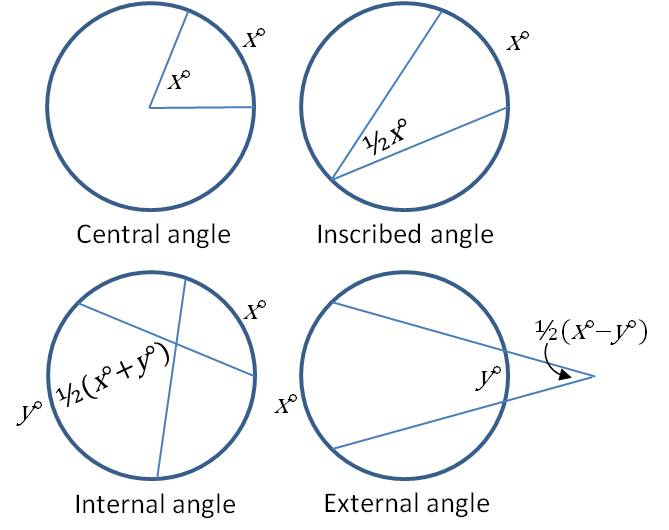## Biconditional statements

When a conditional and its converse are both true, we call this a biconditional. A biconditional means that the hypothesis is true if and only if the conclusion is true. [Some math teachers like to abbreviate ‘if and only if’ as ‘iff’.]

It is also appropriate to call a biconditional statement a definition, because it says the hypothesis and the conclusion imply each other (so they are the same thing).

Here’s an example of a biconditional:

Conditional:  If an angle is a right angle, then it has a measure of 90°.

Converse: If an angle has a measure of 90°, then it is a right angle.

Both of these statements are true, and so we could also call this a definition: A right angle is an angle with a measure of 90°.

## Mastering geometry proofs

A good strategy for completing a proof in geometry is to work backwards. If you are having trouble determining where to start, try going to the end first. Look at the statement you are trying to prove and ask yourself, “What do I need to know in order to prove this statement?” In other words, what would the last line of the proof be? Then figure out what you need in order to prove the next to last line of the proof, and so on.

Here’s an example to show how this works:

Given:$\overline{AE}$ and$\overline{BD}$ bisect each other.
Prove:$\overline{AB} \cong \overline{DE}.$

How do you approach this? The first question to ask yourself is “what do I need to know to prove the two segments are congruent?” The answer: Show that the two triangles are congruent—then the segments will be congruent by CPCTC.

So next, the question becomes “how do I show that the triangles are congruent?” Well, you can show triangles are congruent with SSS, SAS or ASA.

Continuing to work backwards, the next question is “can I prove SSS?” Well, no, because you’re trying to prove two sides are congruent. You can’t use what you’re trying to prove in a congruence proof! So ask the next question: “can I prove SAS?” And the answer is yes!

Because$\overline{AE}$ and$\overline{BD}$ bisect each other,$\overline{AC} \cong \overline{CE}$ and$\overline{BC} \cong \overline{CD}.$    And$\angle{ACB} \cong \angle{DCE}$  because they are vertical angles.

You have simplified the proof to finding two congruent sides and a congruent angle. This leads to SAS which leads to two congruent triangles which leads to the two congruent segments you were trying to prove.

## Finding the missing acute angle in a right triangle

In a right triangle, the two acute angles add up to 90°. If you are trying to find one missing angle, it’s usually easier to find the complement of the other acute angle than to subtract the two known angles from 180°.

Example: Find angle A.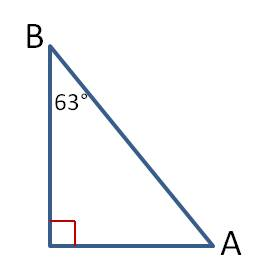Solution: Since this is a right triangle, A and B add up to 90. Therefore, 90 – 63 = 27°.

## Conditional, contrapositive, converse, inverse

A conditional statement and its contrapositive are equivalent; they are either both true or both false. The converse of a conditional and the inverse of a conditional are also equivalent. But a conditional and its converse are not equivalent. When a conditional is true, its converse may be true or false.

Let’s look at some examples:

Conditional 1: If my dog hears the doorbell, then she will bark.

The contrapositive of this statement is If my dog does not bark, then she did not hear the doorbell. Both the conditional and the contrapositve are true.

Conditional 2: If my dog hears the doorbell, then she will moo.

The contrapositive of this statement is If my dog does not moo, then she did not hear the doorbell. Both of these statements are false (and pretty silly).

Here are the converse and inverse of conditional 1:

Converse: If my dog barks, then she heard the doorbell.

Inverse: If my dog did not hear the doorbell, then she will not bark.  These two statements are both false. (My dog will bark for many reasons other than the doorbell.